# Long Mollifiers

Let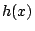be a real polynomial with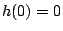, let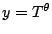for some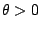, and let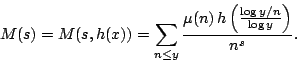The Dirichlet polynomial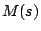is called a mollifier'' of the Riemann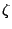-function because it is an approximation to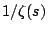, and so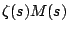should be much better behaved than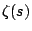near the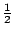-line.

The mean value ofnear the-line is a fundamental tools for studying zeros of the-function. The most general version currently used is the following formula of Conrey, Ghosh, and Gonek [ MR 90h:11077]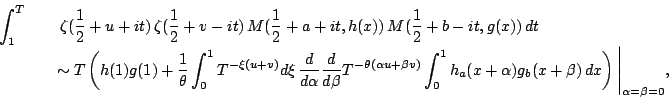where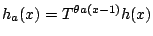, uniformly for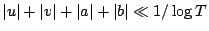. This formula is used in Levinson's method [ MR 58 #27837], and is known to be valid [ MR 90g:11120] for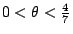. Showing that the formula is valid for large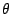is key to having good results.

Farmer [ MR 95a:11076] conjectured that the above formula should remain valid for all. This leads to a conjecture for an integral involving ratios of zeta-functions, which implies the pair-correlation conjecture. See the original paper [ MR 95a:11076] for some additional consequences.

It would be a significant result to prove that the above formula holds for some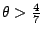. Establishing it for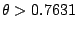would prove that more than half of the zeros of the-function are on the-line. Proving that it holds for allis more-or-less equivalent to the GUE conjecture, because it can be deduced from the formulas for ratios of zeta-functions.

Back to the main index for L-functions and Random Matrix Theory.